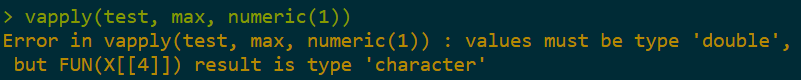Want to share your content on R-bloggers? click here if you have a blog, or here if you don't.In this post we’ll cover the vapply function in R. vapply is generally lesser known than the more popular sapply, lapply, and apply functions. However, it is very useful when you know what data type you’re expecting to apply a function to as it helps to prevent silent errors. Because of this, it can be more advisable to use vapply rather than sapply or lapply.

See more R articles by clicking here

## Examples

Let’s take the following example. Here, we have a list of numeric vectors and we want to get the max value of each vector. That’s simple enough – we can just use sapply and apply the max function for each vector.

```test <- list(a = c(1, 3, 5), b = c(2,4,6), c = c(9,8,7))

sapply(test, max)

```

But what if our list also contains a vector of characters, rather than numeric values? Running the example below gives us the max value of each vector, but coerces the final result to a character vector, which would not be desirable if our code / script is expecting numeric results.

```test\$d <- c("a", "b", "c")

sapply(test, max)

```To get around this, we can use vapply. In this case, we just need to add an extra parameter that specifies the data type expected for the function being applied – i.e. when we apply the max function we expect to be applying it to numeric vectors. Running the code below will result in an error, rather than coercing the final result to a character vector.

```vapply(test, max, numeric(1))

```numeric(1) signifies that we just want individual numeric values. numeric(0) would signify we’re expecting zero-length numeric values.

To adjust the expected data type, we just need to change the third parameter. For example, if we are expecting a list of data frames, we could write:

```vapply(frames, nrow, data.frame(1))

```

Alternatively, the third parameter is called FUN.VALUE, so we could also do this:

```vapply(frames, nrow, FUN.VALUE = data.frame(1))

```

Similar to sapply, vapply ahas a simplify argument so that you can choose rather to simplify the result to a vector (if possible) or a list. By default, simplify is set to TRUE.

```test\$d <- NULL

vapply(test, max, numeric(1), simplify = FALSE)

```

## Conclusion

That’s all for now! Check out online courses here to learn more about R / Python / data science (including my course on web scraping)! To learn about other lesser-known apply functions, see this post.

The post Why you should use vapply in R appeared first on Open Source Automation.